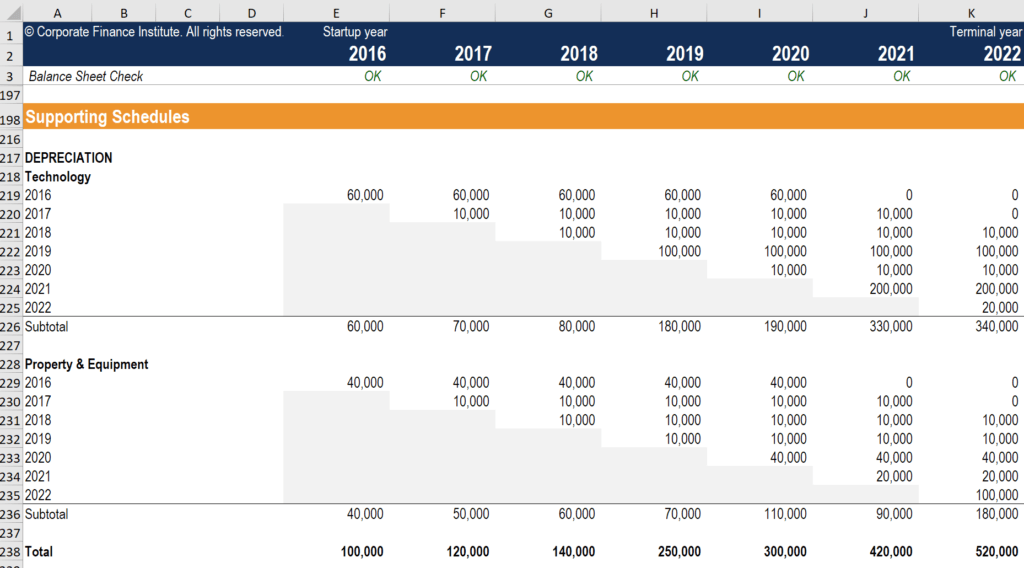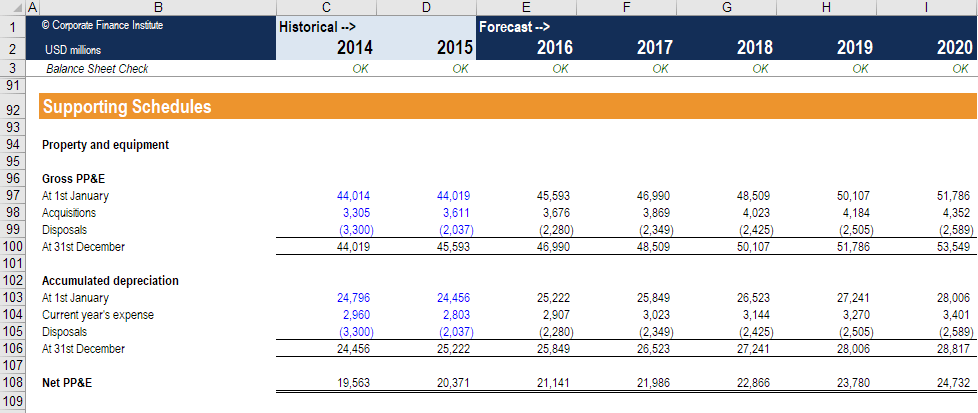# Depreciation Schedule

Calculate depreciation expense with a schedule

## What is a Depreciation Schedule?

A depreciation schedule is required in financial modeling to forecast the value of a company’s fixed assets (balance sheet), depreciation expense (income statement) and capital expenditures (cash flow statement).

Depreciation occurs when an economic asset is used up. This includes different types of property, plant, and equipment (PP&E). As these assets are used, they begin to degrade and lose value. Different assets lose value at different rates, and a depreciation schedule helps outline these differences. The schedule will list the different classes of assets, the type of depreciation method they use, and the cumulative depreciation they have incurred up to that point in time. The depreciation schedule may also include historic and forecast capital expenditures (capex).The screenshot above is an example of 5-year straight-line depreciation, from CFI’s e-commerce financial modeling course.

### Setting up the depreciation schedule

To begin with, create the structure for the depreciation schedule as follows. The first line item to be referenced should be sales revenue. This is because sales revenue is a common driver for both capital expenditure and depreciation expense.

Below this, prepare a section for capital expenditures and reference historical capex from any available periods. Project future capital expenditures by using an appropriate forecasting assumption. Apply intuition to determine the proper forecasting assumption to use from the following:

• Capex as a percentage of sales
• Fixed recurring amount
• Reasonable “hard dollars” that one would expect a company to incur as they operate

### This forecast will depend on the type of operation

If applying the capex as a percentage of sales method, divide capex by sales to find capital expenditure as a percentage of sales. Use these percentages to create an assumption about future capital expenditure as a percentage of sales. Multiply this against projected sales to find a forecast for capital expenditure.

Prepare a section for depreciation expense and reference historical depreciation expense for any available periods. With depreciation expense, there is also room for interpretation on which forecasting assumption to use. Apply judgment based on the industry and business undertaken to select assumptions from the following:

• Depreciation expense as a percentage of CapEx
• Depreciation expense as a percentage of net PP&E
• Depreciation expense as a percentage of sales
• Fixed amount
• Reasonable growth rate

If it seems, in the past, that depreciation expense has remained constant, the company may be using a linear depreciation policy, such as the straight-line depreciation method. In such a case, it is handy to use depreciation expense as a percentage of net PP&E, or to simply roll forward the recurring depreciation amount.

### Summarizing the depreciation schedule

At the bottom of the depreciation schedule, prepare a breakdown of the change in PP&E. This begins with the beginning balance of PP&E, net of accumulated depreciation. From this beginning balance, add capital expenditures, subtract depreciation expense and subtract any sales or write-offs. The final total should be the ending balance of PP&E, already net of accumulated depreciation.At this point, it may be prudent to smooth the projections. If it seems that the trend in the future is too wonky, or the relationship between future capex and depreciation expense becomes dissimilar, consider revisiting the forecasting assumptions for each item. Real estate is a specific industry that requires heavy use of the depreciation schedule.

### More resources

While the three core statements are the backbone of the financial model, the depreciation schedule is one of many supporting schedules that help solidify a model.

CFI is the official provider of the global Financial Modeling & Valuation Analyst (FMVA)™ certification program, designed to help anyone become a world-class financial analyst. To keep advancing your career, the additional resources below will be useful:

• Net working capital schedule
• Debt schedule
• Core Statements
• Financial Modelling Overview

### Financial Modeling Certification

Become a certified Financial Modeling and Valuation Analyst (FMVA)® by completing CFI’s online financial modeling classes!